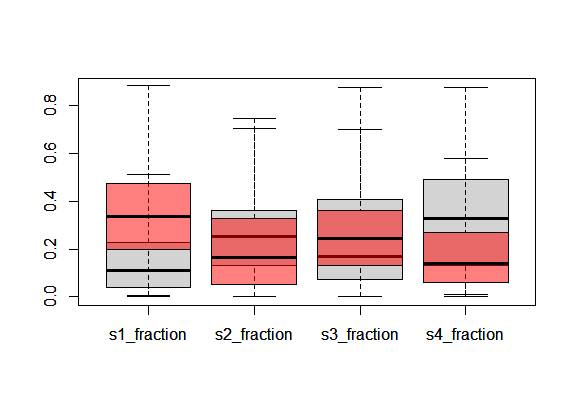# isoWater

## Introduction

isoWater includes two sets of tools. The first supports queries to the Waterisotopes Database (wiDB, https://waterisotopesDB.org) to identify and download data. The second uses Bayesian estimation to infer information about the origin of a water sample based on its stable isotope ratios.

## wiDB Queries

Three functions are available to streamline queries to the wiDB. wiDB_values() is designed to expose the list of terms used in the database’s categorical metadata fields, helping the user design other queries:

wiDB_values(c("types", "countries"))
## $types ##  "Beer" "Bottled" "Canal" "Cave_drip" ##  "Cloud_or_fog" "Firn_core" "Ground" "Ice_core" ##  "Lake" "Leaf" "Milk" "Mine" ##  "Ocean" "Precipitation" "Rime" "River_or_stream" ##  "Snow_pit" "Soil" "Spring" "Sprinkler" ##  "Stem" "Tap" "Vapor" ## ##$countries
##    "AD" "AE" "AF" "AL" "AM" "AO" "AQ" "AR" "AT" "AU" "BA" "BB" "BD" "BE" "BF"
##   "BG" "BH" "BI" "BJ" "BM" "BO" "BR" "BW" "BY" "BZ" "CA" "CD" "CF" "CH" "CL"
##   "CM" "CN" "CO" "CR" "CU" "CV" "CY" "CZ" "DE" "DJ" "DK" "DO" "DZ" "EC" "EE"
##   "EG" "ES" "ET" "FI" "FK" "FM" "FO" "FR" "GA" "GB" "GE" "GF" "GH" "GI" "GL"
##   "GR" "GT" "GU" "GY" "HK" "HN" "HR" "HU" "ID" "IE" "IL" "IN" "IO" "IQ" "IR"
##   "IS" "IT" "JO" "JP" "KE" "KG" "KH" "KI" "KR" "KW" "KY" "KZ" "LA" "LB" "LK"
##   "LS" "LT" "LU" "LV" "LY" "MA" "MC" "MD" "MG" "MK" "ML" "MM" "MN" "MT" "MU"
##  "MX" "MY" "MZ" "NA" "NE" "NG" "NI" "NL" "NO" "NP" "NZ" "OM" "PA" "PE" "PF"
##  "PG" "PH" "PK" "PL" "PR" "PS" "PT" "PW" "PY" "QA" "RE" "RO" "RS" "RU" "SA"
##  "SC" "SD" "SE" "SG" "SH" "SI" "SJ" "SK" "SN" "ST" "SV" "SY" "SZ" "TD" "TH"
##  "TJ" "TN" "TR" "TW" "TZ" "UA" "UG" "UK" "UM" "US" "UY" "UZ" "VE" "VN" "WS"
##  "ZA" "ZM" "ZW"

wiDB_sites() queries the database and returns a data.frame containing the latitude and longitude coordinates for all sites matching the provided query criteria. This supports quick screening of data availability, for example:

ls = wiDB_sites(countries = "US", types = "Lake")
ps = wiDB_sites(countries = "US", types = "Precipitation")
omar = par("mar")
par(mar = c(4, 4, 1, 1))
plot(ls[, 2:1], xlim = c(-125, -68), ylim = c(25, 50))
points(ps[, 2:1], col = "red")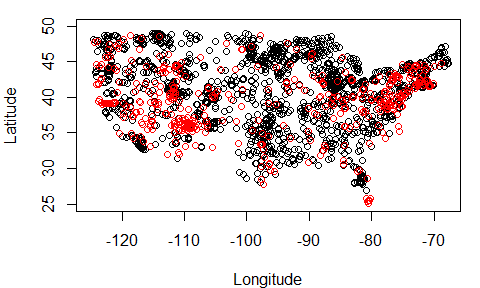par(mar = omar)

Now we can refine our query and request isotope data using the function wiDB_data(). We will focus in on the area around Ames, Iowa, where the sites query showed both lake and precipitation data.

ld = wiDB_data(minLat = 41, maxLat = 42, minLong = -94, maxLong = -93, types = "Lake")
pd = wiDB_data(minLat = 41, maxLat = 42, minLong = -94, maxLong = -93, types = "Precipitation")
ld$data ## Site_ID Site_Name Latitude Longitude Elevation Sample_ID Type ## 1 NLA06608-2036 South Banner Lake 41.43839 -93.55097 238.24 710256 Lake ## 2 NLA06608-0008 Lake Ahquabi 41.29211 -93.59040 261.56 710288 Lake ## 3 NLA06608-0008 Lake Ahquabi 41.29211 -93.59040 261.56 710971 Lake ## 4 NLA06608-2056 Badger Creek Lake 41.46950 -93.92037 278.75 710972 Lake ## Start_Date Start_Time_Zone Collection_Date Collection_Time_Zone Phase ## 1 NA NA 2007-07-11 00:00:00 NA Liquid ## 2 NA NA 2007-07-12 00:00:00 NA Liquid ## 3 NA NA 2007-08-22 00:00:00 NA Liquid ## 4 NA NA 2007-08-22 00:00:00 NA Liquid ## Depth_meters Sample_Comments d2H d18O D17O d2H_Analytical_SD ## 1 0 NA -15.56189 -0.5534326 NA NA ## 2 0 NA -25.35876 -2.0486228 NA NA ## 3 0 NA -20.81503 -1.9292234 NA NA ## 4 0 NA -27.77034 -3.3816347 NA NA ## d18O_Analytical_SD D17O_Analytical_SD WI_Analysis_Source Project_ID ## 1 NA NA EPA_Corvallis 10 ## 2 NA NA EPA_Corvallis 10 ## 3 NA NA EPA_Corvallis 10 ## 4 NA NA EPA_Corvallis 10 pd$projects
##   Project_ID Contact_Name Contact_Email
## 1         31           NA            NA
##                                                                                                                               Citation
## 1 W.W. Simpkins, Isotopic composition of precipitation in central Iowa, Journal of Hydrology, Volume 172, Issue 1, 1995, Pages 185-207
##                                            URL                Project_Name
## 1 https://doi.org/10.1016/0022-1694(95)02694-K Iqbal, 2008; Simpkins, 1995

Notice that the object returned by the data function is a named list that includes the requested data and a summary of the projects (data sources) from which those data are derived. See the documentation for this function for details, and always remember to cite any data you use!

Let’s look at the data a little more closely:

omar = par("mar")
par(mar = c(5, 5, 1, 1))
plot(pd$data$d18O, pd$data$d2H, xlab = expression(delta^{18}*"O"),
ylab = expression(delta^{2}*"H"))
abline(10, 8)
points(ld$data$d18O, ld$data$d2H, col = "red")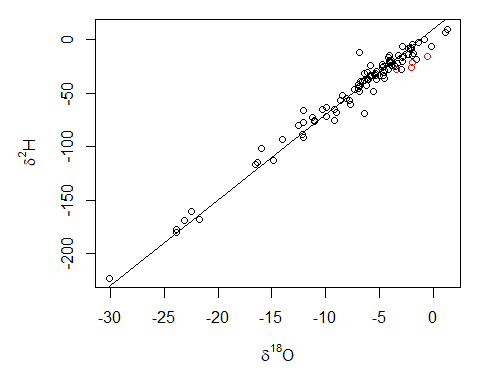par(mar = omar)

It appears that the precipitation values vary widely and cluster around the Global Meteoric Water Line, and the lake values are relatively high and somewhat below the GMWL, suggesting that they may be somewhat evaporatively enriched.

## Meteoric Water Line source

What if we wanted to ‘correct’ for the isotopic effects of evaporation, giving sample values that could be compared directly with one or more unevaporated sources. In other words, based on isotopic data for a water sample and parameters describing a local meteoric water line (MWL), estimate the isotope ratios of the sample’s source prior to evaporation. If you have data you’d like to use to constrain the MWL, like we do here, the mwl function will produce a data object describing the line:

#extract the precipitation H and O isotope values
HO = data.frame(pd$data$d2H, pd$data$d18O)
MWL = mwl(HO)
## Warning in mwl(HO): Missing values removed from HO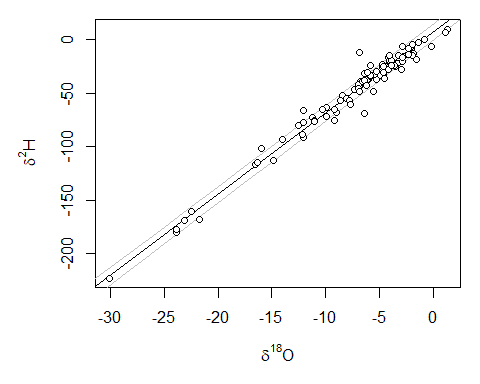If you don’t have local data for a MWL, the function mwlSource() (see below) has parameters for the Global Meteoric Water Line embedded as a default.

Now bundle the data for your water sample as an “iso” data object using the function iso(). Note that isoWater uses a bivariate normal distribution to describe all water sample values; thus the parameters in an iso object include the H and O isotope values, their 1 standard deviation uncertainties, and the error covariance for H and O. For measured sample values the covariance might be expected to be zero (the default value in iso()), but for many values (e.g., potential sample or source values estimated from multiple measurements) the H and O error covariance will be substantial.

#we will analyze one of our lake water samples, and assume realistic
#values of analytical uncertainty and no error covariance
obs = iso(ld$data$d2H, ld$data$d18O, 0.5, 0.1)

Lastly, we need to provide a prior distribution for the evaporation line slope, which is represented as a univariate normal distribution with mean and standard deviation parameters. These can be tricky to estimate, but there are number of papers out there that provide guidance, as well as some tools and data products that might help.

#assumed value that is reasonable for lake water evaporation in this
#location/climate
slope = c(5.2, 0.3)

With our inputs prepared, we can run the analysis using mwlSource().

mwls1 = mwlSource(obs, MWL, slope, ngens = 5e3)
## module glm loaded
mwls1$summary ## mean sd 2.5% 25% 50% ## E 7.7081650 2.6704816 3.393547 5.588384 7.5122408 ## S 5.2109529 0.2965399 4.624784 5.011905 5.2160622 ## deviance -0.3104334 2.0198979 -2.269598 -1.744046 -0.9416131 ## source_d18O -8.2618865 2.6627993 -13.562813 -10.321231 -8.0674442 ## source_d2H -55.9021590 14.7631205 -85.769483 -67.618816 -53.8783381 ## 75% 97.5% Rhat n.eff ## E 9.7859836 13.033213 1.968886 5 ## S 5.4139821 5.771030 1.036471 66 ## deviance 0.4738062 4.990815 1.001033 7500 ## source_d18O -6.1571968 -3.972886 1.828618 6 ## source_d2H -44.1705487 -32.879424 1.800157 6 The summary object returned by the function provides two statistics that can be used to assess convergence (Rhat and effective sample size), in addition to summary statistics for the posterior distribution. For the relatively small chain lengths run in these examples we don’t expect strong convergence. The function’s output also includes an object containing all posterior samples (isoWater functions thin the posterior to 2500 samples per chain by default), which we can plot as a trace plot to visually assess burn-in and convergence: plot(mwls1$results$source_d2H[1:2500], type = "l", ylim = range(mwls1$results$source_d2H)) lines(mwls1$results$source_d2H[2501:5000], col=2) lines(mwls1$results$source_d2H[5001:7500], col=3)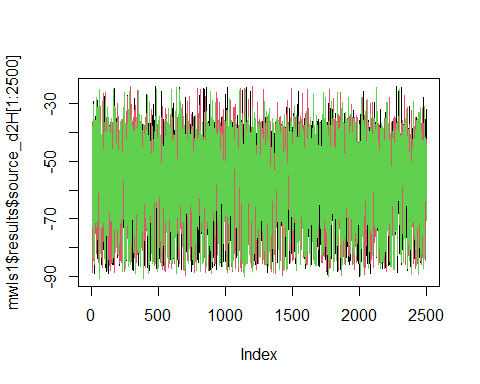The MWL model uses one of two line statistics to constrain the source water prior distribution. The default, shown above, uses the MWL prediction interval as the prior, appropriate if the source is best represented as a single sample of the type used to define the MWL. Alternatively, the argument stype = 2 can be provided to use the confidence interval as the prior constraint, appropriate for samples where the source is best represented as a mixture of many samples of the type used to define the MWL. You can see that this makes a substantial difference in the resulting posterior distribution, so make sure you’re using the right statistics for your problem: mwls2 = mwlSource(obs, MWL, slope, stype = 2, ngens = 5e3) omar = par("mar") par(mar = c(5, 5, 1, 1)) plot(HO[, 2:1], xlim = c(-20, 0), ylim = c(-140, 0), xlab = expression(delta^{18}*"O"), ylab = expression(delta^{2}*"H")) abline(MWL, MWL) points(obs[2:1], col = "red") points(mwls1$results$source_d18O, mwls1$results$source_d2H, col = "blue") points(mwls2$results$source_d18O, mwls2$results$source_d2H, col = "green")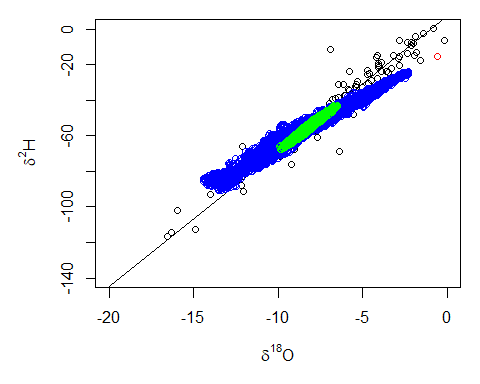par(mar = omar) ## Mixture source What if instead of just back-correcting our sample values for evaporation, we wanted to explicitly assess the relative contribution of different water sources to the sample? In other words, based on isotope values for two or more potential water sources, what is the mixture of sources that contributed to the sample? The mixSource() function works similarly to mwlSource(), but requires different arguments to define the mixing model source priors. Values for all sources are provided in a single iso object: #prep our data - we'll average the precipitation values by quarter q = quarters(as.POSIXct(pd$data$Collection_Date)) qu = sort(unique(q)) ql = length(qu) pd_q = data.frame("H" = numeric(ql), "O" = numeric(ql), "Hsd" = numeric(ql), "Osd" = numeric(ql), "HOc" = numeric(ql)) for(i in seq_along(qu)){ pd_q$H[i] = mean(pd$data$d2H[q == qu[i]], na.rm = TRUE)
pd_q$O[i] = mean(pd$data$d18O[q == qu[i]], na.rm = TRUE) pd_q$Hsd[i] = sd(pd$data$d2H[q == qu[i]], na.rm = TRUE)
pd_q$Osd[i] = sd(pd$data$d18O[q == qu[i]], na.rm = TRUE) pd_q$HOc[i] = cov(pd$data$d18O[q == qu[i]], pd$data$d2H[q == qu[i]],
use = "pairwise.complete.obs")
}
pd_q
##            H          O      Hsd      Osd       HOc
## 1 -111.36000 -15.634667 59.49320 7.434212 439.68889
## 2  -32.91821  -4.784643 24.80235 3.257371  77.32559
## 3  -26.05879  -4.285000 19.06960 2.374869  44.77920
## 4  -54.53600  -9.051429 36.48143 4.546960 163.92950
#make the iso object, providing the stats calculated above
sources = iso(pd_q$H, pd_q$O, pd_q$Hsd, pd_q$Osd, pd_q$HOc) Now we can run the analysis; let’s use the parallel option (also available in mwlSource()) to speed this up and run slightly longer chains: mixs1 = mixSource(obs, sources, slope, ngens = 2e4, ncores = 2) mixs1$summary
##                     mean        sd          2.5%          25%         50%
## E              8.2848892 1.5288373   5.524627826   7.18940669   8.1552191
## S              5.2317270 0.2741893   4.676632206   5.05122839   5.2410935
## deviance      -0.3271234 1.9914124  -2.270293531  -1.74138273  -0.9415298
## fracs       0.1652494 0.1716670   0.004283999   0.03914978   0.1094585
## fracs       0.2609011 0.1642916   0.010482061   0.13273786   0.2509954
## fracs       0.2512432 0.2187772   0.008025836   0.07174243   0.1693958
## fracs       0.3226063 0.2010874   0.026730664   0.13203708   0.3261766
## mixture_d18O  -8.8379821 1.5191582 -11.924260039  -9.87403038  -8.7104230
## mixture_d2H  -59.0938037 9.2688091 -77.806589447 -65.19115841 -58.1292540
##                      75%       97.5%     Rhat n.eff
## E              9.3256715  11.3804263 1.183500    13
## S              5.4191895   5.7499351 1.011605   140
## deviance       0.4295615   5.0132555 1.003275  2700
## fracs       0.2294817   0.6837321 1.250029    10
## fracs       0.3609446   0.6411943 1.003913   480
## fracs       0.4065147   0.7580442 1.504315     6
## fracs       0.4906114   0.6944143 1.017223   150
## mixture_d18O  -7.7603033  -6.1050989 1.203828    12
## mixture_d2H  -52.4716499 -43.0713659 1.174259    13

Again, we’re a long way from having a strongly converged simulation in which the posterior is likely to be a representative sample of the population parameters, but the initial ‘hint’ is that the lake sample values are consistent with a sub-equal source contribution from precipitation in each calendar quarter. Notice, too, that the evaporation-corrected sample values (‘mixture_d18O’ and ‘mixture_d2H’) are broadly similar to the equivalent values we obtained with the MWL model, above.

The default options use a prior that weights each source evenly, but if we have other information we can use the arguments prior and shp to prescribe different distributions. Values in prior are relative, so for example if we think Q1 is likely to have contributed twice as much as the other seasons…this may or may not have a strong impact on the results, depending on the strength of the constraints provided by the isotopic data:

mixs2 = mixSource(obs, sources, slope, prior = c(2, 1, 1, 1), ngens = 2e4, ncores = 2)
boxplot(mixs1$results[, 3:6], outline = FALSE) boxplot(mixs2$results[, 3:6], add = TRUE, col = rgb(1, 0, 0, 0.5),
outline = FALSE)